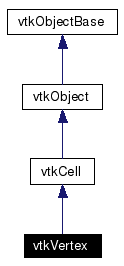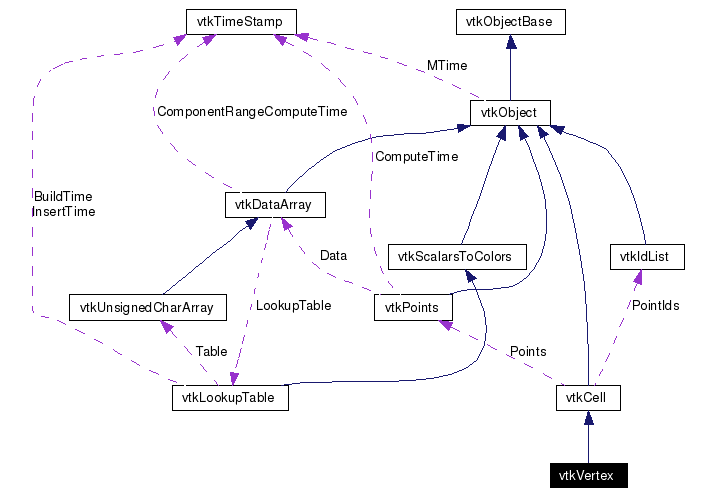# vtkVertex Class Reference

`#include <vtkVertex.h>`

Inheritance diagram for vtkVertex:[legend]
Collaboration diagram for vtkVertex:[legend]
List of all members.

## Detailed Description

a cell that represents a 3D point

vtkVertex is a concrete implementation of vtkCell to represent a 3D point.

Examples:
vtkVertex (Examples)
Tests:
vtkVertex (Tests)

Definition at line 32 of file vtkVertex.h.

## Public Types

typedef vtkCell Superclass

## Public Member Functions

virtual const char * GetClassName ()
virtual int IsA (const char *type)
void PrintSelf (ostream &os, vtkIndent indent)
int GetCellType ()
int GetCellDimension ()
int GetNumberOfEdges ()
int GetNumberOfFaces ()
vtkCellGetEdge (int)
vtkCellGetFace (int)
void Clip (double value, vtkDataArray *cellScalars, vtkPointLocator *locator, vtkCellArray *pts, vtkPointData *inPd, vtkPointData *outPd, vtkCellData *inCd, vtkIdType cellId, vtkCellData *outCd, int insideOut)
int EvaluatePosition (double x, double *closestPoint, int &subId, double pcoords, double &dist2, double *weights)
void EvaluateLocation (int &subId, double pcoords, double x, double *weights)
virtual double * GetParametricCoords ()
int CellBoundary (int subId, double pcoords, vtkIdList *pts)
void Contour (double value, vtkDataArray *cellScalars, vtkPointLocator *locator, vtkCellArray *verts1, vtkCellArray *lines, vtkCellArray *verts2, vtkPointData *inPd, vtkPointData *outPd, vtkCellData *inCd, vtkIdType cellId, vtkCellData *outCd)
int GetParametricCenter (double pcoords)
int IntersectWithLine (double p1, double p2, double tol, double &t, double x, double pcoords, int &subId)
int Triangulate (int index, vtkIdList *ptIds, vtkPoints *pts)
void Derivatives (int subId, double pcoords, double *values, int dim, double *derivs)

## Static Public Member Functions

static vtkVertexNew ()
static int IsTypeOf (const char *type)
static vtkVertexSafeDownCast (vtkObject *o)
static void InterpolationFunctions (double pcoords, double weights)

vtkVertex ()
~vtkVertex ()

## Member Typedef Documentation

 typedef vtkCell vtkVertex::Superclass
 Reimplemented from vtkCell. Definition at line 36 of file vtkVertex.h.

## Constructor & Destructor Documentation

 vtkVertex::vtkVertex ( ) ` [protected]`

 vtkVertex::~vtkVertex ( ) ` [inline, protected]`
 Definition at line 108 of file vtkVertex.h.

## Member Function Documentation

 static vtkVertex* vtkVertex::New ( ) ` [static]`
 Create an object with Debug turned off, modified time initialized to zero, and reference counting on. Reimplemented from vtkObject.

 virtual const char* vtkVertex::GetClassName ( ) ` [virtual]`
 Reimplemented from vtkCell.

 static int vtkVertex::IsTypeOf ( const char * type ) ` [static]`
 Return 1 if this class type is the same type of (or a subclass of) the named class. Returns 0 otherwise. This method works in combination with vtkTypeRevisionMacro found in vtkSetGet.h. Reimplemented from vtkCell.

 virtual int vtkVertex::IsA ( const char * type ) ` [virtual]`
 Return 1 if this class is the same type of (or a subclass of) the named class. Returns 0 otherwise. This method works in combination with vtkTypeRevisionMacro found in vtkSetGet.h. Reimplemented from vtkCell.

 static vtkVertex* vtkVertex::SafeDownCast ( vtkObject * o ) ` [static]`
 Reimplemented from vtkCell.

 void vtkVertex::PrintSelf ( ostream & os, vtkIndent indent ) ` [virtual]`
 Methods invoked by print to print information about the object including superclasses. Typically not called by the user (use Print() instead) but used in the hierarchical print process to combine the output of several classes. Reimplemented from vtkCell.

 int vtkVertex::GetCellType ( ) ` [inline, virtual]`
 See the vtkCell API for descriptions of these methods. Implements vtkCell. Definition at line 43 of file vtkVertex.h. References VTK_VERTEX.

 int vtkVertex::GetCellDimension ( ) ` [inline, virtual]`
 Return the topological dimensional of the cell (0,1,2, or 3). Implements vtkCell. Definition at line 44 of file vtkVertex.h.

 int vtkVertex::GetNumberOfEdges ( ) ` [inline, virtual]`
 Return the number of edges in the cell. Implements vtkCell. Definition at line 45 of file vtkVertex.h.

 int vtkVertex::GetNumberOfFaces ( ) ` [inline, virtual]`
 Return the number of faces in the cell. Implements vtkCell. Definition at line 46 of file vtkVertex.h.

 vtkCell* vtkVertex::GetEdge ( int ) ` [inline, virtual]`
 Return the edge cell from the edgeId of the cell. Implements vtkCell. Definition at line 47 of file vtkVertex.h.

 vtkCell* vtkVertex::GetFace ( int ) ` [inline, virtual]`
 Return the face cell from the faceId of the cell. Implements vtkCell. Definition at line 48 of file vtkVertex.h.

 void vtkVertex::Clip ( double value, vtkDataArray * cellScalars, vtkPointLocator * locator, vtkCellArray * pts, vtkPointData * inPd, vtkPointData * outPd, vtkCellData * inCd, vtkIdType cellId, vtkCellData * outCd, int insideOut ) ` [virtual]`
 Cut (or clip) the cell based on the input cellScalars and the specified value. The output of the clip operation will be one or more cells of the same topological dimension as the original cell. The flag insideOut controls what part of the cell is considered inside - normally cell points whose scalar value is greater than "value" are considered inside. If insideOut is on, this is reversed. Also, if the output cell data is non-NULL, the cell data from the clipped cell is passed to the generated contouring primitives. (Note: the CopyAllocate() method must be invoked on both the output cell and point data. The cellId refers to the cell from which the cell data is copied.) Implements vtkCell.

 int vtkVertex::EvaluatePosition ( double x, double * closestPoint, int & subId, double pcoords, double & dist2, double * weights ) ` [virtual]`
 Given a point x return inside(=1) or outside(=0) cell; evaluate parametric coordinates, sub-cell id (!=0 only if cell is composite), distance squared of point x to cell (in particular, the sub-cell indicated), closest point on cell to x (unless closestPoint is null, in which case, the closest point and dist2 are not found), and interpolation weights in cell. (The number of weights is equal to the number of points defining the cell). Note: on rare occasions a -1 is returned from the method. This means that numerical error has occurred and all data returned from this method should be ignored. Also, inside/outside is determine parametrically. That is, a point is inside if it satisfies parametric limits. This can cause problems for cells of topological dimension 2 or less, since a point in 3D can project onto the cell within parametric limits but be "far" from the cell. Thus the value dist2 may be checked to determine true in/out. Implements vtkCell.

 void vtkVertex::EvaluateLocation ( int & subId, double pcoords, double x, double * weights ) ` [virtual]`
 Determine global coordinate (x) from subId and parametric coordinates. Also returns interpolation weights. (The number of weights is equal to the number of points in the cell.) Implements vtkCell.

 virtual double* vtkVertex::GetParametricCoords ( ) ` [virtual]`
 Return a contiguous array of parametric coordinates of the points defining this cell. In other words, (px,py,pz, px,py,pz, etc..) The coordinates are ordered consistent with the definition of the point ordering for the cell. This method returns a non-NULL pointer when the cell is a primary type (i.e., IsPrimaryCell() is true). Note that 3D parametric coordinates are returned no matter what the topological dimension of the cell. Reimplemented from vtkCell.

 int vtkVertex::CellBoundary ( int subId, double pcoords, vtkIdList * pts ) ` [virtual]`
 Given parametric coordinates of a point, return the closest cell boundary, and whether the point is inside or outside of the cell. The cell boundary is defined by a list of points (pts) that specify a vertex (1D cell). If the return value of the method is != 0, then the point is inside the cell. Implements vtkCell.

 void vtkVertex::Contour ( double value, vtkDataArray * cellScalars, vtkPointLocator * locator, vtkCellArray * verts1, vtkCellArray * lines, vtkCellArray * verts2, vtkPointData * inPd, vtkPointData * outPd, vtkCellData * inCd, vtkIdType cellId, vtkCellData * outCd ) ` [virtual]`
 Generate contouring primitives. The scalar list cellScalars are scalar values at each cell point. The point locator is essentially a points list that merges points as they are inserted (i.e., prevents duplicates). Implements vtkCell.

 int vtkVertex::GetParametricCenter ( double pcoords ) ` [inline, virtual]`
 Return the center of the triangle in parametric coordinates. Reimplemented from vtkCell. Definition at line 116 of file vtkVertex.h.

 int vtkVertex::IntersectWithLine ( double p1, double p2, double tol, double & t, double x, double pcoords, int & subId ) ` [virtual]`
 Intersect with a ray. Return parametric coordinates (both line and cell) and global intersection coordinates, given ray definition and tolerance. The method returns non-zero value if intersection occurs. Implements vtkCell.

 int vtkVertex::Triangulate ( int index, vtkIdList * ptIds, vtkPoints * pts ) ` [virtual]`
 Triangulate the vertex. This method fills pts and ptIds with information from the only point in the vertex. Implements vtkCell.

 void vtkVertex::Derivatives ( int subId, double pcoords, double * values, int dim, double * derivs ) ` [virtual]`
 Get the derivative of the vertex. Returns (0.0, 0.0, 0.0) for all dimensions. Implements vtkCell.

 static void vtkVertex::InterpolationFunctions ( double pcoords, double weights ) ` [static]`
 Vertex specific methods.

The documentation for this class was generated from the following file:

Generated on Tue Jan 22 00:27:58 2008 for VTK by1.4.3-20050530